Courses

# Properties of Metals, Stress & Strain Civil Engineering (CE) Notes | EduRev

## Topic wise GATE Past Year Papers for Civil Engineering

Created by: Gate Gurus

## Civil Engineering (CE) : Properties of Metals, Stress & Strain Civil Engineering (CE) Notes | EduRev

The document Properties of Metals, Stress & Strain Civil Engineering (CE) Notes | EduRev is a part of the Civil Engineering (CE) Course Topic wise GATE Past Year Papers for Civil Engineering.
All you need of Civil Engineering (CE) at this link: Civil Engineering (CE)

Q.1 A plate in equilibrium is subjected to uniform stresses along its edges with magnitude σxx= 30 MPa and σyy = 50 MPa as shown in the figure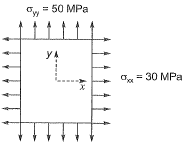The Young’s modulus of the material is 2 x 1011 N/m2 and the Poisson’s ratio is 0.3. If σzz is negligibly small and assumed to be zero, then the strain εzz is               [2018 : 2 Marks, Set-I]

(a) -120 x 10-6
(b) -60 x 10-6
(c) 0.0
(d) 120 x 10-6
Solution:Q.2 A 2 m long, axially loaded mild steel rod of 8 mm diameter exhibits the load-displacement (P-δ) behavior as shown in the figure.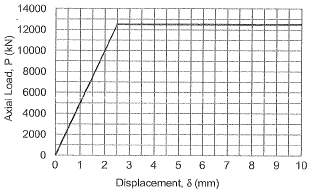Assume the yield stress of steel as 250 MPa. The complementary strain energy (in N-mm) stored in the bar up to its linear elastic behaviour will be_____.[2017 : 2 Marks, Set-II]
Solution: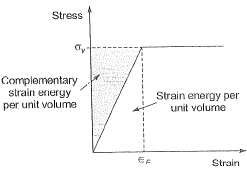Elastic strain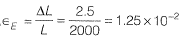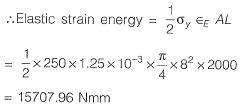Note: For linear elastic material both complementary energy and strain energy is same.
OR
By considering given graph in question, between Axial Load and Displacement the solution will be as follows: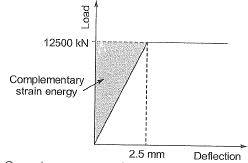Complementary stain energy.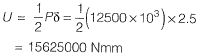It means there seems some error in the given data.

Q.3 In a material under a state of plane strain, a 10 x 10 mm square centered at a point gets deformed as shown in the figure.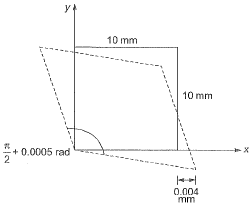If the shear strain γxy at this point is expressed as 0.001 k (in rad), the value of k is    [2017 : 1 Mark, Set-II]
(a) 0.50

(b) 0.25
(c) -0.25
(d) -0.50
Ans.
(D)
Solution:
According to the sign convention,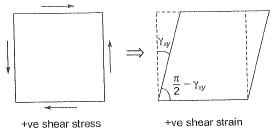In question since angle has been increase therefore shear strain should be negative.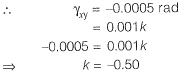Q.4 Consider the stepped bar made with a linear elastic material and subjected to an axial load of 1 kN, as shown in the figure.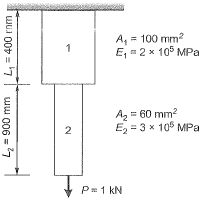Segments 1 and 2 have cross-sectional area of 100 mm2 and 60 mm2. Young’s modulus of 2 x 105 MPa and 3 x 105 MPa, and length of 400 mm and 900 mm, respectively. The strain energy (in N-mm upto one decimal place) in the bar due to the axial load i s _________.    [2017 : 2 Marks, Set-I]
Solution: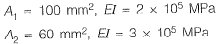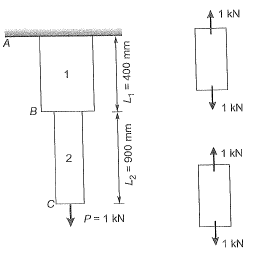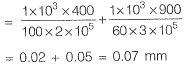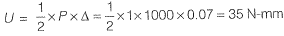Q.5 An elastic bar of length L, uniform cross-sectional area A, coefficient of thermal expansion α, and Young’s modulus Eis fixed at the two ends. The temperature of the bar is increased by T, resulting in an axial stress σ. Keeping all other parameters unchanged, if the length of the bar is doubled, the axial stress would be    [2017 : 1 Mark, Set-I]
(a) σ
(b) 2 σ
(c) 0.5 σ
(d) 0.25 σ
Ans. (A)
Solution: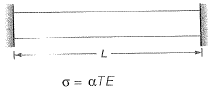∴ Length have no effect on thermal stress.
∴ Axial stress is only ‘σ’.

Q.6 An elastic isotropic body is in a hydrostatic state of stress as shown in the figure. For no change in the volume to occur, what should be its Poisson’s ratio?    [2016 : 2 Marks, Set-II]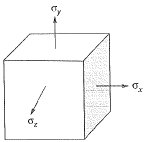(a) 0.00
(b) 0.25
(c) 0.50
(d) 1.00
Ans. (C)
Solution:
Volumetric strain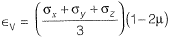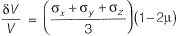As ΔV = 0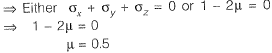Q.7 A tapered circular rod of diameter varying from 20 mm to 10 mm is connected to another uniform circular rod of diameter 10 mm as shown in the following figure. Both bars are made of same material with the modulus of elasticity, E = 2 x 105 MPa. When subjected to a load P = 30πkN, the deflection at point A is ___ mm.    [2015 : 2 Marks, Set-I]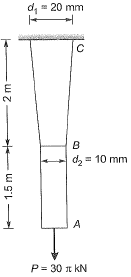Solution: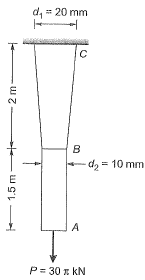Total elongation,
AB is uniform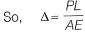BC is tapere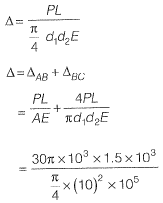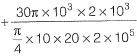= (9 + 6) mm = 15 mm

Q.8 For the state of stresses (in MPa) shown in the figure below, the maximum shear stress (in MPa) is _____.    [2014 : 2 Marks, Set-II]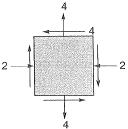Solution: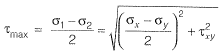= 5.0 MPa

Q.9 The values of axial stress (σ) in kN/m2, bending moment (M) in kNm, and shear force (V) in kN acting at point P for the arrangement shown in the figure are respectively    [2014 : 2 Marks, Set-II]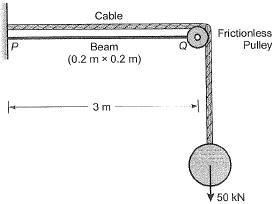(a) 1000, 75 and 25
(b) 1250, 150 and 50
(c) 1500, 225 and 75
(d) 1750, 300 and 100
Ans.
(B)
Solution: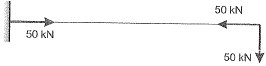Bending moment = 50 x 3 = 150 kNm
Shear force = 50kN

Q.10 A box of weight 100 kN shown in the figure is to be lifted without swinging. If all forces are coplanar, the magnitude and direction (θ) of the force (F) with respect to x-axis should be    [2014 : 2 Marks, Set-I]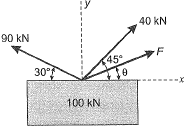(a) F= 56.389 kN and θ = 28.280
(b) F = - 56.389 kN and θ = - 28.280
(c) F = 9.055 kN and θ = 1.4140
(d) F = - 9.055 kN and θ = - 1.4140
Ans.
(A)
Solution: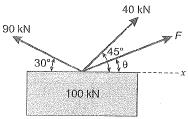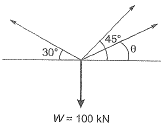For no swinging force should be balanced i.e.,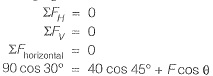or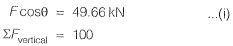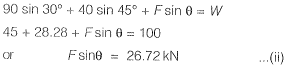Dividina ea. (ii) by eq. (i) we get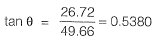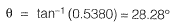Substituting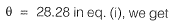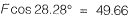or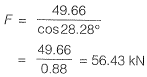Q.11 Creep strains are [2013 : 1 Mark]
(b) caused due to live load only
(c) caused due to cyclic load only
Ans.
(A)
Solution:
Creep strains occur with time at a constant level of stress. So they occur due to permanent load i.e., Dead Load.

Q.12 The Poisson’s ratio is defined as [2012 : 1 Mark]
(a)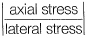(b)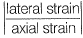(c)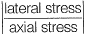(d)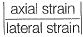Ans.
(B)
Solution: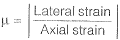Q.13 A rigid beam is hinged at one end and supported on linear elastic springs (both having a stiffness of 'K) at points T and ‘2’, and an inclined load acts at ‘2’, as shown.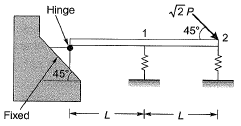If the load Pequals 100 kN, which of the following options represents forces P1 and P2 in the springs at points ‘1’ and ‘27'? [2011 : 2 Marks]
(a) R1 = 20 kN and R2 = 40 kN
(b) R1 = 50 kN and R2 = 50 kN
(c) R1 = 30 kN and R2 = 60 kN
(d) R1 = 40 kN and R2 = 80 kN
Ans.
(D)
Solution: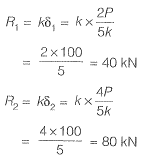Q.14 A rigid beam is hinged at one end and supported on linear elastic springs (both having a stiffness of 'K) at points T and ‘2’, and an inclined load acts at ‘2’, as shown.Which of the following options represents the deflections δ1 and δ2 at points ‘Y and ‘2’?    [2011 : 2 Marks]
(a)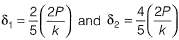(b)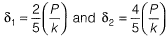(c)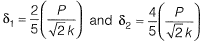(d)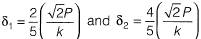Ans.
(B)
Solution:
The free diagram of the beam is shown below,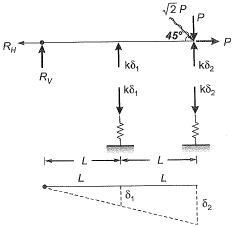From similar triangles, we get,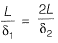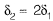...(i)
Taking moments about hinge, we get,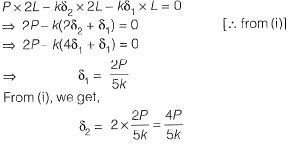Q.15 The number of independent elastic constants for a linear elastic isotropic and homo-geneous material is   [2010 : 1 Mark]
(a) 4
(b) 3
(c) 2
(d) 1
Ans.
(C)
Solution: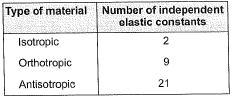Offer running on EduRev: Apply code STAYHOME200 to get INR 200 off on our premium plan EduRev Infinity!

,

,

,

,

,

,

,

,

,

,

,

,

,

,

,

,

,

,

,

,

,

,

,

,

;